Python

# Return Multiple Values from A Python Function

The function is used in any programming language to run a specific block of code multiple times when require and organize the code properly. Sometimes, this requires reading the return value from the function for the programming purposes. The return value of the function is stored in a variable. Python functions can return both single and multiple values. This tutorial shows how multiple values can be returned from Python functions with multiple variables, objects, tuples, lists, and dictionaries.

### Example 1: Return multiple values from the function using multiple variables

If you want to return only a few variables from the Python function, then it is better to define these variables to store the multiple return values from the multiple variables, objects, tuples, lists, and dictionaries using the function. In this example, three variables are declared in the script to store three return values. The multiVarFunc() function is used to take three input values and return the values to the variables dept_name, total_std and total_fac.

#!/usr/bin/env python3

# Define function to return multiple variables
def multiVarFunc():
# Take a string data
dept = input("Enter department name: ")
# Take a numeric data
stdnum = int(input("Enter the number of total students: "))
# Take a numeric data
facnum = int(input("Enter the number of total faculties: "))
# Return multiple variables
return dept,stdnum,facnum;

# Call the function and store the return values in three variables
dept_name, total_std, total_fac = multiVarFunc()
# Print the formatted output of the return values
print("\nDepartment:%s\nTotal students:%d\nTotal faculties:%d" %(dept_name,total_std,
total_fac))

Output

Three values are taken as the inputs, and the output values are printed after formatting.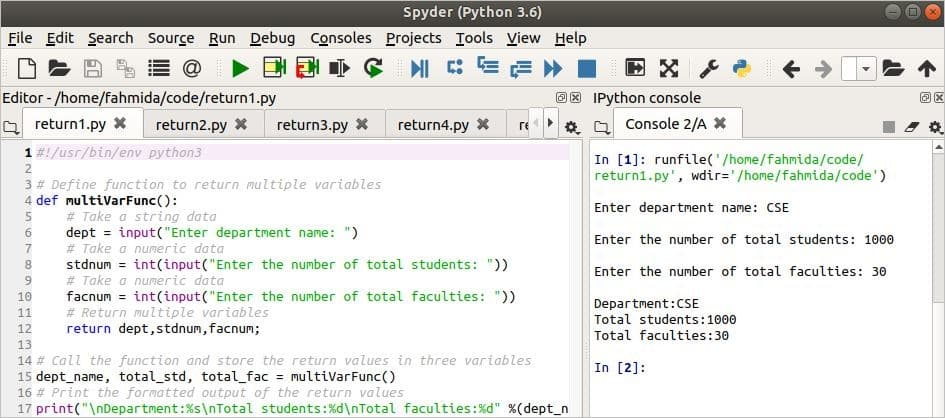### Example 2: Return multiple values from the function using the tuple

The following script shows how to use a tuple to return multiple values from a function. If you want to return many values from the function, then this is a better option. Here, the tupleFunc() function is used to take four input values from the user and return the values as a tuple to the caller. The return values will be stored in a tuple variable named tupleVar and the values will be printed later.

#!/usr/bin/env python3

# Define function to return multiple variables
def tupleFunc():
# Take a string data
stdID = input("Enter the student Id:")
# Take a string data
stdName = input("Enter the student name: ")
# Take a integer data
stdBatch = int(input("Enter the batch No: "))
# Take a float data
stdCGPA = float(input("Enter the CGPA: "))
# Return multiple variables as a tuple
return (stdID,stdName,stdBatch,stdCGPA)

# Call the function and store the return values in a tuple
tupleVar = tupleFunc()
# Print the formatted output of the tuple
print("\n ID:%s\n Name:%s\n Batch:%d\n CGPA:%4.2f" %(tupleVar,tupleVar,tupleVar,
tupleVar))

Output

The four input values are taken as the input and formatted output values are then printed.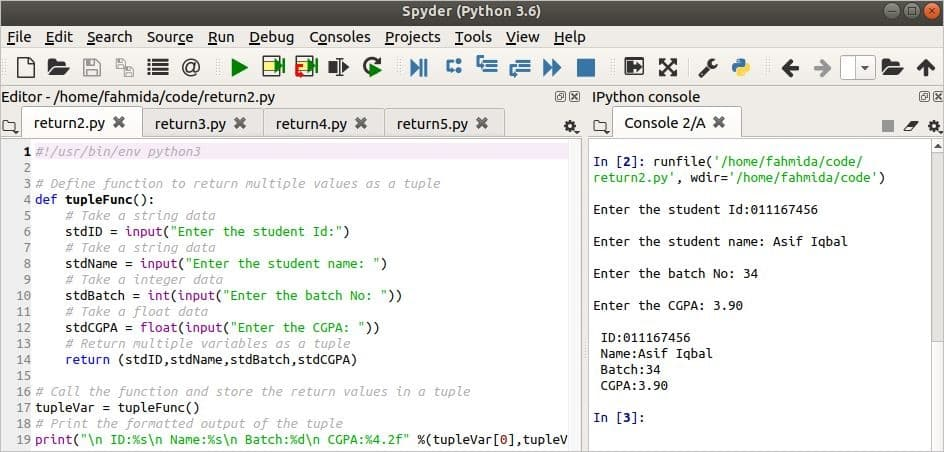### Example 3: Return multiple values from the function using the list

The following script shows how to use a list to return multiple values from a function. This is another option to return many values from a function. The listFunc() function is used in the script to take two integer numbers from the user and calculate the addition, subtraction, multiplication, and division of these numbers. Next, these four results are returned as a list from the function. The list variable, listVar is used to store the return values and print the values.

#!/usr/bin/env python3

# Define function to return multiple values as a list
def listFunc():
# Take a numeric data
number1 = float(input("Enter any number:"))
# Take a numeric data
number2 = float(input("Enter any number:"))

subtraction = number1 - number2
multiplication = number1 * number2
division = number1 / number2

# Return multiple variables as a list
return [number1, number2, addition, subtraction, multiplication, division]

# Call the function and store the return values in a tuple
listVar = listFunc()
# Print the formatted output of the list data
print("\n%5.2f + %5.2f = %5.2f" %(listVar, listVar, listVar))
print("%5.2f - %5.2f = %5.2f" %(listVar, listVar, listVar))
print("%5.2f x %5.2f = %5.2f" %(listVar, listVar, listVar))
print("%5.2f / %5.2f = %5.2f" %(listVar, listVar, listVar))

Output

After taking two numbers, 67 and 23.7, the following output will appear.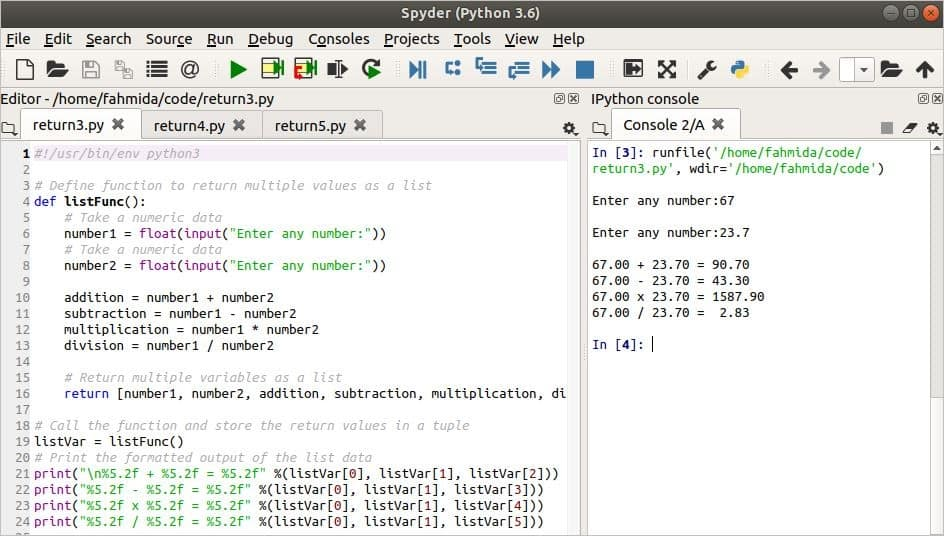### Example 4: Return multiple values from the function using the dictionary

The following script shows how to use a dictionary to return multiple values from a function. This is another option to return many values from a function. A dictionary object variable named dictVar is declared inside the function. Three values are assigned to the variable and return the dicVar to the caller. Next, the dictionary values are printed.

#!/usr/bin/env python3
# Define function to return the multiple values as a dictionary
def dictFunc():
# Declare a dictionary variable
dictVar = dict();
# Assign some values
dictVar['name'] = "Kelli Ali"
dictVar['age']   = 46
dictVar['profession'] = 'Singer'
# Return the dictionary as return values
return dictVar

# Call the function and store the return values in a dictionary variable
dictValues = dictFunc()
# Print the dictionary values
print("The values of dictionary are:\n", dictValues)

Output

The following output will appear after running the script.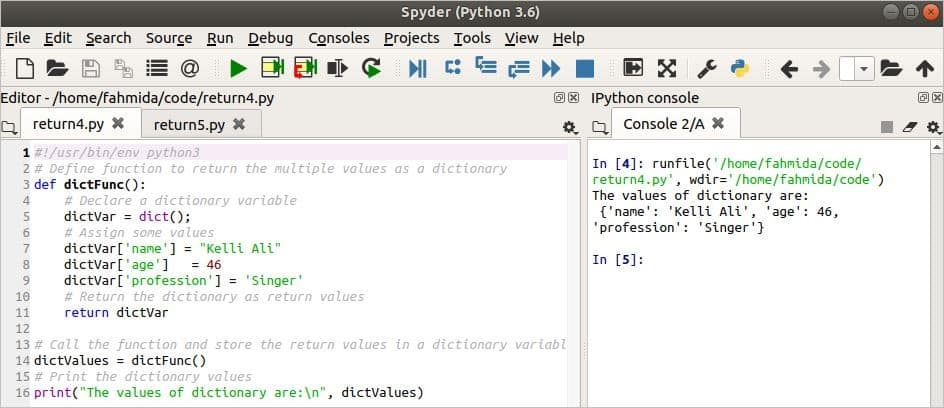### Example 5: Return multiple values from the function using object

A class is used with a function to return multiple values as an object from the function in the following script. When the objFunc() function calls, the function initializes the object from the Employees class and returns the object to the caller. Next, the property values of the object will be printed.

#!/usr/bin/env python3

# Define the class to intialize the object
class Employees:
def __init__(self):
self.name = "Mosarof Karim"
self.post = "Manager"
self.salary = 50000

# Define the function to return values as an object
def objFunc():
return Employees()

# Call the function to set the object variable
objVar = objFunc()

# Print the formatted output
print("\n Employee Name:", objVar.name,"\n","Post:",objVar.post,"\n","Salary:",
objVar.salary)

Output

The following output will appear after running the script.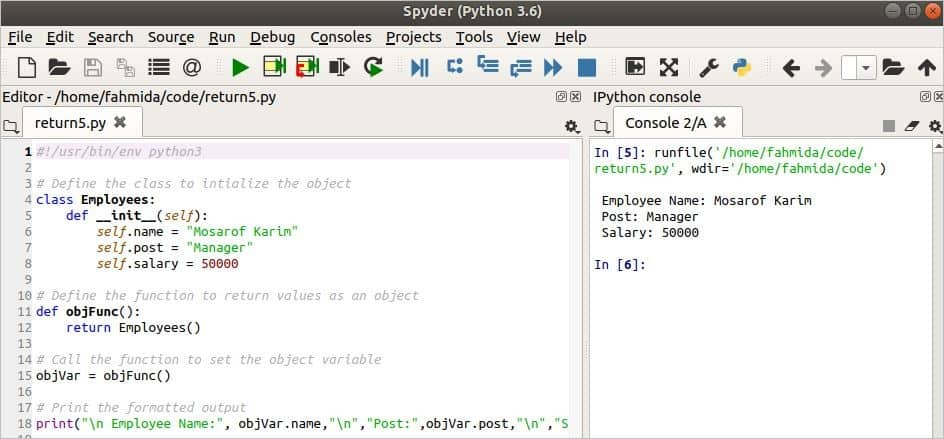### Conclusion

Multiple return values from a function can be used in the script for various purposes. This tutorial showed some of the different ways of returning multiple values from a function in Python.